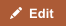A million is 106, or 1,000,000. A billion is one thousand million, or 1,000,000,000 (109). This is the common usage in English-speaking countries and is called the short scale. Countries in continental Europe and Latin America use the long scale where a billion is a million millions (1012).

The word billion originated from French word bi- (“two”) + -illion; i.e. a million million. It was first coined by Jehan Adam in 1475 as by-million and then rendered as byllion by Nicolas Chuquet in 1484.

Million originated from the Italian milione, from the Latin mille + the augmentative suffix -one.

Comparison chart

Billion versus Million comparison chartBillionMillion
Power of 10 10 to the 9th power (10^9) 10 to the 6th power (10^6)
Number 1,000,000,000 1,000,000

Magnitude of the difference

The magnitude of difference between billion and million can be illustrated with this example of the time scale:

• A million seconds is 12 days.
• A billion seconds is 31 years.
• A trillion seconds is 31,688 years.

The video further compares the three numbers

Other large numbers

• Million=1,000,000
• Billion=1,000,000,000
• Trillion=1,000,000,000,000
• Quintillion=1,000,000,000,000,000,000
• Sextillion=1,000,000,000,000,000,000,000,000.
• Nonillion=1,000,000,000,000,000,000,000,000,000,000
• CENTILLION=1 followed by 303 zeros